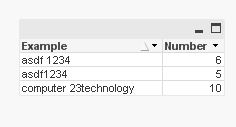# QlikView App Development

Discussion Board for collaboration related to QlikView App Development.

HighlightedNot applicable

## How to find the position of first numerical value?

Hi All,

I have a below requirement to be accomplished,

In a string I need to find the position of first numerical value from left.

Examples:

'asdf1234',

'asdf 1234',

'computer 23technology'

From the above strings I need to get the output of 5,6,10 respectively, which are positions for the first numeric from left.

The above are the example strings and it could vary in any manner.

Thanks & Regards,

Karthikeyan A R.

Tags (7)
1 Solution

Accepted SolutionsMVP

## Re: How to find the position of first numerical value?Data:

Load *, FindOneOf(Example, '1234567890')  as Number

inline [

Example

asdf1234

asdf 1234

computer 23technology

];

7 Replies
Valued Contributor II

## Re: How to find the position of first numerical value?

Try this

Data:
Load *, index(Example,left(keepchar(Example,0123456789),1)) as tmp1 inline [
Example
asdf1234
asdf 1234
computer 23technology
]
;

flipside

Honored Contributor III

## Re: How to find the position of first numerical value?

Try this.

=Index('computer 23technology',2)

=
Index('asdf 1234',1)

=
Index('asdf1234',1)

Esteemed Contributor

## Re: How to find the position of first numerical value?

Hi,

from the internal help I gather that you can pass several characters to look for to the INDEX() function? Then you could write like

>>> INDEX([field], '0123456789')  <<<

and that should return the position of the first occurence of any of these, no?

Give it a try.

HTH

Best regards,

DataNibblerNot applicable

## Re: How to find the position of first numerical value?

But the strings with numeric will be dynamic. We cannot exactly say whether the number is 1 or 2 or 3.

So first we need to find the first number in string from left and then its position.MVP

## Re: How to find the position of first numerical value?Data:

Load *, FindOneOf(Example, '1234567890')  as Number

inline [

Example

asdf1234

asdf 1234

computer 23technology

];Not applicable

## Re: How to find the position of first numerical value?

Hi,

I have tried the above but it is not working as I expected.

The below function works,

Index('text01234567890','0123456789')

But not with this:  Index('text0123','0123456789')

ThanksNot applicable

## Re: How to find the position of first numerical value?

Perfect!!!

It worksThanks much.